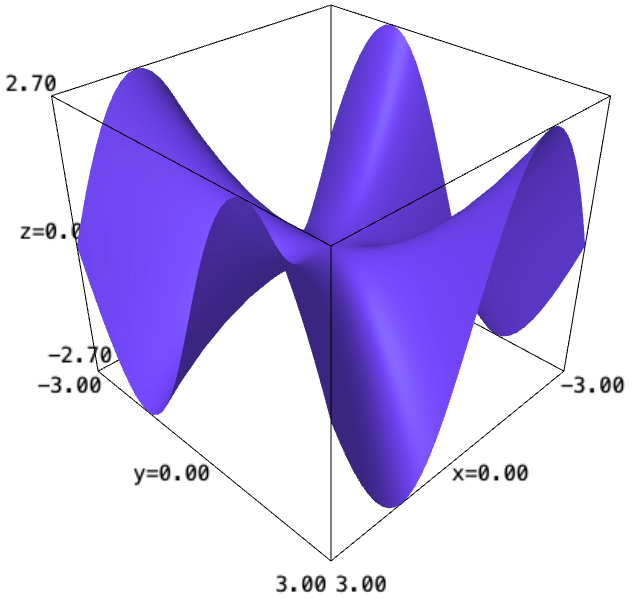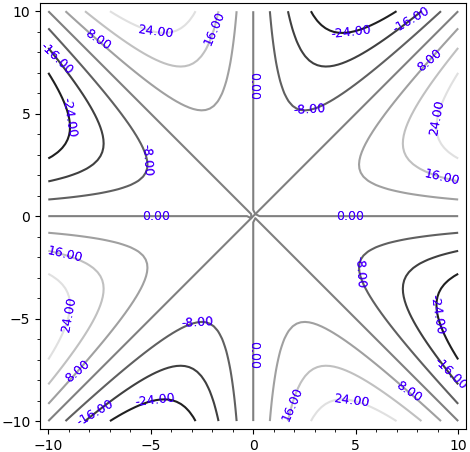# How do I define the function to be 0 at x=0?

Struggling first-year undergrad here... sorry if this question seems stupid.

I want to graph the 3d and contour plots of f(x,y)=x*y*(x^2-y^2))/(x^2+y^2). Since the function is undefined at 0 I need to also define the function to be 0 at x=0.

Here's what I wrote

x, y = var('x y')
plot3d((x*y*(x^2-y^2))/(x^2+y^2), (-3, 3), (-3, 3))contour_plot((x*y*(x^2-y^2))/(x^2+y^2), (-10, 10), (-10, 10), fill=0, labels=40)The contour plot looks a bit funky, there's a big cross made by two lines at the origin which I suppose is because the function is undefined at 0.

edit retag close merge delete

Sort by » oldest newest most voted

Sage doesn't (yet) have symbolic boolean functions (but see Trac#31911...). But since :

sage: f(0,y)
0
sage: f(x,0)
0


we can write :

sage: h(x,y)=cases([(x==0, 0), (y==0, 0), (True, f(x,y))])


which is defined at 0.

But plotting $h$ has the same problem as plotting $f$ : the "big cross", is a plotting artefact, resulting from the way Sage computes the numerical values of points to be plotted.

The workaround would be to compute explicit expression for your level curves and plot them. This is left to the reader as an exercise ;-))...

HTH,

EDIT : In your case, this might not be necessary ; writing your function in polar coordinates being enough to get an expression with no singularities at $(0,0)$ :

sage: var("r, theta")
(r, theta)
sage: g(r,theta)=f(x,y).subs({x:r*cos(theta), y:r*sin(theta)}).simplify_full() ; g
(r, theta) |--> (2*r^2*cos(theta)^3 - r^2*cos(theta))*sin(theta)
sage: g(0, theta)
0


HTH,

more